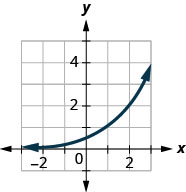$$\newcommand{\id}{\mathrm{id}}$$ $$\newcommand{\Span}{\mathrm{span}}$$ $$\newcommand{\kernel}{\mathrm{null}\,}$$ $$\newcommand{\range}{\mathrm{range}\,}$$ $$\newcommand{\RealPart}{\mathrm{Re}}$$ $$\newcommand{\ImaginaryPart}{\mathrm{Im}}$$ $$\newcommand{\Argument}{\mathrm{Arg}}$$ $$\newcommand{\norm}{\| #1 \|}$$ $$\newcommand{\inner}{\langle #1, #2 \rangle}$$ $$\newcommand{\Span}{\mathrm{span}}$$

# 10.3E: Exercises

•• OpenStax
• OpenStax
$$\newcommand{\vecs}{\overset { \rightharpoonup} {\mathbf{#1}} }$$ $$\newcommand{\vecd}{\overset{-\!-\!\rightharpoonup}{\vphantom{a}\smash {#1}}}$$$$\newcommand{\id}{\mathrm{id}}$$ $$\newcommand{\Span}{\mathrm{span}}$$ $$\newcommand{\kernel}{\mathrm{null}\,}$$ $$\newcommand{\range}{\mathrm{range}\,}$$ $$\newcommand{\RealPart}{\mathrm{Re}}$$ $$\newcommand{\ImaginaryPart}{\mathrm{Im}}$$ $$\newcommand{\Argument}{\mathrm{Arg}}$$ $$\newcommand{\norm}{\| #1 \|}$$ $$\newcommand{\inner}{\langle #1, #2 \rangle}$$ $$\newcommand{\Span}{\mathrm{span}}$$ $$\newcommand{\id}{\mathrm{id}}$$ $$\newcommand{\Span}{\mathrm{span}}$$ $$\newcommand{\kernel}{\mathrm{null}\,}$$ $$\newcommand{\range}{\mathrm{range}\,}$$ $$\newcommand{\RealPart}{\mathrm{Re}}$$ $$\newcommand{\ImaginaryPart}{\mathrm{Im}}$$ $$\newcommand{\Argument}{\mathrm{Arg}}$$ $$\newcommand{\norm}{\| #1 \|}$$ $$\newcommand{\inner}{\langle #1, #2 \rangle}$$ $$\newcommand{\Span}{\mathrm{span}}$$

### Practice Makes Perfect

##### Exercise $$\PageIndex{17}$$ Graph Exponential Functions

In the following exercises, graph each exponential function.

1. $$f(x)=2^{x}$$
2. $$g(x)=3^{x}$$
3. $$f(x)=6^{x}$$
4. $$g(x)=7^{x}$$
5. $$f(x)=(1.5)^{x}$$
6. $$g(x)=(2.5)^{x}$$
7. $$f(x)=\left(\frac{1}{2}\right)^{x}$$
8. $$g(x)=\left(\frac{1}{3}\right)^{x}$$
9. $$f(x)=\left(\frac{1}{6}\right)^{x}$$
10. $$g(x)=\left(\frac{1}{7}\right)^{x}$$
11. $$f(x)=(0.4)^{x}$$
12. $$g(x)=(0.6)^{x}$$

1.

3.

5.

7.

9.

11.

##### Exercise $$\PageIndex{18}$$ Graph Exponential Functions

In the following exercises, graph each function in the same coordinate system.

1. $$f(x)=4^{x}, g(x)=4^{x-1}$$
2. $$f(x)=3^{x}, g(x)=3^{x-1}$$
3. $$f(x)=2^{x}, g(x)=2^{x-2}$$
4. $$f(x)=2^{x}, g(x)=2^{x+2}$$
5. $$f(x)=3^{x}, g(x)=3^{x}+2$$
6. $$f(x)=4^{x}, g(x)=4^{x}+2$$
7. $$f(x)=2^{x}, g(x)=2^{x}+1$$
8. $$f(x)=2^{x}, g(x)=2^{x}-1$$

1.

3.

5.

7.

##### Exercise $$\PageIndex{19}$$ Graph Exponential Functions

In the following exercises, graph each exponential function.

1. $$f(x)=3^{x+2}$$
2. $$f(x)=3^{x-2}$$
3. $$f(x)=2^{x}+3$$
4. $$f(x)=2^{x}-3$$
5. $$f(x)=\left(\frac{1}{2}\right)^{x-4}$$
6. $$f(x)=\left(\frac{1}{2}\right)^{x}-3$$
7. $$f(x)=e^{x}+1$$
8. $$f(x)=e^{x-2}$$
9. $$f(x)=-2^{x}$$
10. $$f(x)=2^{-x-1}-1$$

1.

3.

5.

7.

9.

##### Exercise $$\PageIndex{20}$$ Solve Exponential Equations

In the following exercises, solve each equation.

1. $$2^{3 x-8}=16$$
2. $$2^{2 x-3}=32$$
3. $$3^{x+3}=9$$
4. $$3^{x^{2}}=81$$
5. $$4^{x^{2}}=4$$
6. $$4^{x}=32$$
7. $$4^{x+2}=64$$
8. $$4^{x+3}=16$$
9. $$2^{x^{2}+2 x}=\frac{1}{2}$$
10. $$3^{x^{2}-2 x}=\frac{1}{3}$$
11. $$e^{3 x} \cdot e^{4}=e^{10}$$
12. $$e^{2 x} \cdot e^{3}=e^{9}$$
13. $$\frac{e^{x^{2}}}{e^{2}}=e^{x}$$
14. $$\frac{e^{x^{2}}}{e^{3}}=e^{2 x}$$

1. $$x=4$$

3. $$x=-1$$

5. $$x=-1, x=1$$

7. $$x=1$$

9. $$x=-1$$

11. $$x=2$$

13. $$x=-1, x=2$$

##### Exercise $$\PageIndex{21}$$ Solve Exponential Equations

In the following exercises, match the graphs to one of the following functions:

1. $$2^{x}$$
2. $$2^{x+1}$$
3. $$2^{x-1}$$
4. $$2^{x}+2$$
5. $$2^{x}-2$$
6. $$3^{x}$$

1.Figure 10.2.37

2.Figure 10.2.38

3.Figure 10.2.39

4.Figure 10.2.40

5.Figure 10.2.41

6.Figure 10.2.42

1. f

3. a

5. e

##### Exercise $$\PageIndex{22}$$ Use Exponential Models in Applications

In the following exercises, use an exponential model to solve.

1. Edgar accumulated $$$5,000$$ in credit card debt. If the interest rate is $$20$$% per year, and he does not make any payments for $$2$$ years, how much will he owe on this debt in $$2$$ years by each method of compounding? 1. compound quarterly 2. compound monthly 3. compound continuously 2. Cynthia invested$$$12,000$$ in a savings account. If the interest rate is $$6$$%, how much will be in the account in $$10$$ years by each method of compounding?
1. compound quarterly
2. compound monthly
3. compound continuously
3. Rochelle deposits $$$5,000$$ in an IRA. What will be the value of her investment in $$25$$ years if the investment is earning $$8$$% per year and is compounded continuously? 4. Nazerhy deposits$$$8,000$$ in a certificate of deposit. The annual interest rate is $$6$$% and the interest will be compounded quarterly. How much will the certificate be worth in $$10$$ years?
5. A researcher at the Center for Disease Control and Prevention is studying the growth of a bacteria. He starts his experiment with $$100$$ of the bacteria that grows at a rate of $$6$$% per hour. He will check on the bacteria every $$8$$ hours. How many bacteria will he find in $$8$$ hours?
6. A biologist is observing the growth pattern of a virus. She starts with $$50$$ of the virus that grows at a rate of $$20$$% per hour. She will check on the virus in $$24$$ hours. How many viruses will she find?
7. In the last ten years the population of Indonesia has grown at a rate of $$1.12$$% per year to $$258,316,051$$. If this rate continues, what will be the population in $$10$$ more years?
8. In the last ten years the population of Brazil has grown at a rate of $$0.9$$% per year to $$205,823,665$$. If this rate continues, what will be the population in $$10$$ more years?

1.

1. $$$7,387.28$$ 2.$$$7,434.57$$
3. $$$7,459.12$$ 3.$$$36,945.28$$

5. $$223$$ bacteria

7. $$288,929,825$$

##### Exercise $$\PageIndex{23}$$ Writing Exercises
1. Explain how you can distinguish between exponential functions and polynomial functions.
2. Compare and contrast the graphs of $$y=x^{2}$$ and $$y=2^{x}$$.
3. What happens to an exponential function as the values of $$x$$ decreases? Will the graph ever cross the $$x$$-axis? Explain.